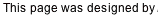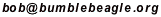## An N-1 hint NxN Du-Sum-Oh exists for any N

A few months after posting my proof (below), I was made aware of an earlier discovery of an 8-hint 9x9 which without much difficulty could be extended to an N-1 proof. It apparently was an outcome of the "Match 2001" competition between Russian and Ukrainian puzzlers. A diagram of that puzzle is shown at diogen.h1.ru/match2001.html. It is the red, green, and blue diagram labled P3 about 70% of the way down the page. It is a simple matter to extend that diagram for any N, and to place the hints so that there is no more than one in any row, column, or region.

As in my construction below, the key to the proof is that the layout permits only one latin square (considering permutations of the symbols as identical), and that is the trivial one in which each symbol occupies one of the toroidal diagonals.

Thanks to Cihan Altay for making me aware of that diagram.

My Proof
(originally posted Jan/9/2006)

Start with a grid partitioned like the one below. Hopefully it's apparent how to extend this to any NxN. Region 1 contains the top three cells in the left column, and the leftmost N-2 along the top row. Region 2 contains the rest of the top row, and the middle N-2 cells of the second row. Region 3 contains all but the top cell of the rightmost column, and the two rightmost cells on the bottom row. The remaining regions layer in as shown.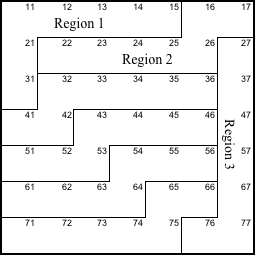It turns out only one latin square is compatible with this grid, except for the N! orderings of the symbols. To show this, I'll start with the symbol at 76, which I will call B. B at 76 forces B at 17 and 65, and then up the diagonal, 54, 43, ... 32. And from there a B must also go in 21.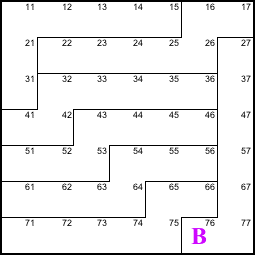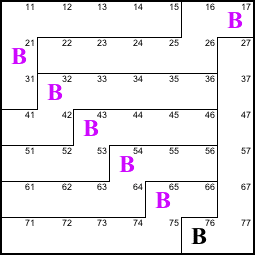Now if I place a C at 31, it percolates down the diagonal to 75. It must also appear at 16 (because row 1 needs a C) and 27.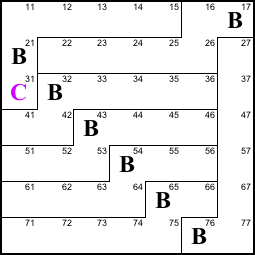Continuing, I place a D at 41 and it percolates down the diagonal to 74. It then hops to the top at 15 and percolates down that diagonal to 37.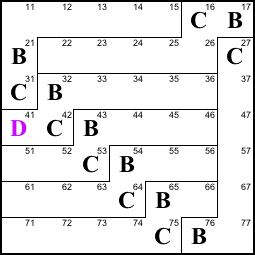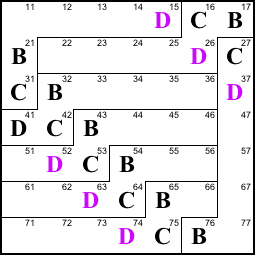At this point you probably get the idea. We put symbol E at 51, F at 61, and so on. Each forces itself along an entire toroidal diagonal. We can do this all the way to the bottom of the first column.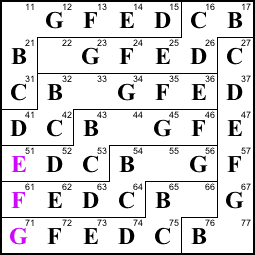After that, the only empty cells remaining are on the main diagonal, and these must all contain whatever symbol remains (e.g. "A").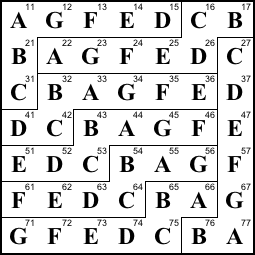So there's only one latin square that fits this grid, for any size N. All we have to do is provide one hint on each of N-1 diagonals, in any pattern we like, and the puzzle has a unique solution. Here's one for N=7.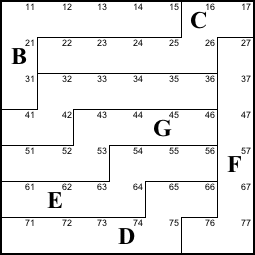The rule doesn't produce interesting puzzles, but at least it provides a constructive proof that for any N there exists a uniquely solvable N-1 hint NxN du-sum-oh (a.k.a. squiggly sudoku, jigsaw sudoku, etc.). And solvable with simple logic, to boot.

Update
(posted Mar/26/2006 and May/20/2006)

The above proof does not guarantee that the hints can be provided in the traditional dusumoh arrangement-- no two hints in the same row, column, or region. Shortly after my original post, John Dalbec showed me how this can be done.

For odd N, the hints lie on a line of constant slope -½, treating the grid as a torus. This is in fact the way I had laid out the hints in the 7x7 example above, but below I include the unused A-clue in gray. This rule works if N is odd because row R, with 1<=R<=N, will contain a clue in column C=2R-3 mod N (with the caveat that we consider column 0 to be N). The columns are distinct because 2 has an inverse modulo odd N. The diagonals are distinct because row R contains its hint on diagonal R-3 (where diagonal 0 contains 11..77). Note that we can remove any one of these hints.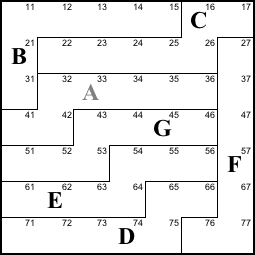John elaborates:
It strikes me that I didn't explain my numbering of the regions. I started with regions 1, 2, and 3 as you labeled them and then I numbered the remaining regions 4..N from top to bottom. I just noticed that you had left the remaining regions unnumbered in your diagram.

This means that region R (4 <= R <= N) contains the squares (R-1,R-2)...(R-1,N-1) and (R,1)...(R,R-2), where (R,C) indicates the square in row R and column C. Since the squares (R,R-1)...(R,N-1) are contained in region R+1 (3 <= R <= N-1), it follows that the square (R,C) (4 <= R <= N-1) belongs to

 region R if 1 <= C <= R-2, region R+1 if R-1 <= C <= N-1, and region 3 if C = N.

The same trichotomy applies when R = 3 except that region R is replaced by region 1. The trichotomy becomes a dichotomy when R = N because region R+1 is replaced by region 3.

We consider the position of the hint in each row and show that these hints lie in distinct regions. The hint in row 1 is in column N-1. The hints in rows 2...(N+3)/2 lie in column 2R-3, where R is the row number. The hints in rows (N+5)/2...N lie in column 2R-N-3.

If 3 <= R <= (N+1)/2, then R-1 <= R <= (C = 2R-3) <= N-2 <= N-1, so the hint in row R belongs to region R+1. If (N+5)/2 <= R <= N, then 1 <= 2 <= (C = 2R-N-3) <= R-3 <= R-2, so the hint in row R belongs to region R. We summarize the hint locations in the following table:

 Row Column Region 1 N-1 2 2 1 1 3...(N+1)/2 2R-3 R+1 (4...(N+3)/2) (N+3)/2 N 3 (N+5)/2...N 2R-N-3 R ((N+5)/2...N)

Thus we can see that each hint is in its own region.

For even N, the hints lie along two adjacent lines of slope -½ (see below). In some of these pairs we use the right letter as a hint; in others we use the left letter. The unused letters are shown in gray.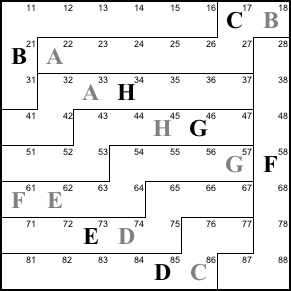Back to Bob’s main Du-Sum-Oh page.